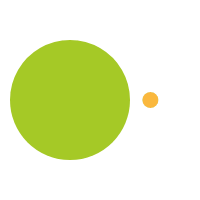# Algebraic Thinking and Valentines

February 8, 2013

No matter what grade level or which curriculum standard is in place, patterns are a crucial aspect of math learning. They are a unifying theme- from creating separate Piles of yellow and green Blocks to understanding the role of “y” in a multi-variable equation. In fact, there are patterns everywhere we look – in shapes, time, language, desk arrangements, buildings, calendars, etc.

But we’ll keep it to mathematical patterns for now. Specifically- number patterns. Number patterns are important because they allow students to make predictions and generalizations, which helps them generate solutions to broader and more complicated problems. Recognizing patterns in numbers is a precursor to recognizing patterns in functions, which are simply rules that define the relationships between various quantities (learner.org).

And so- with moms standing in the aisles of Halmark, wavering between “my little pony” and “care bears” 100-pack valentine cards, let’s work on a Valentine-Card Number-Pattern Math-Problem!

If there are 24 students in class and each student gives one valentine to every other student, how many valentines will be given?

Every grade level can work on this problem, because there are many different ways to get the answer. For younger students, simplify by asking them to start with a smaller number- 3, perhaps- and count or draw the relationships. With 3 students there would be 6 valentines:

A – B

A – C

B – A

B – C

C –A

C –B

How about with 4 students? Create a chart showing the pattern as the number of students increases.

More advanced students can use multiplication to find the answer. With 3 students, each student would hand out two valentines. 3 students x 2 valentines = 6. What about with 4 students? 8? 24?

Bring it to life! Ask your students to each bring one valentine for every other student in class. Have them distribute them, then count them and add them up. Did it work?

A more complicated version of the question can be asked of older students with valentine hugsinstead of valentine cards. If there are 24 students in class and every student hugs every other student once, how many hugs will be given?

Ask them to start by indicating the pattern-

A hugs B

A hugs C

B hugs C

For a classroom of 3 students, there would be 3 hugs. (notice we did not include C hugs B, because that hug has already been counted)

What is the difference between the cards and the hugs? Why?

Ask them to find the formula in the pattern, and explain how they got there. Since they don’t give valentines to themselves, for any number of students, n, each student gives out one less valentine than there are students. That gives us n(n-1), but stopping there would double-count the hugs (because B hugs C is the same as C hugs B). So we divide by two: n(n-1)/2

In a class of 24 students, there would be 24(24-1)/2 or 276 hugs!

Happy Valentine’s Day!